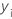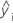# Methods and formulas for the goodness-of-fit statistics for Stability Study for random batches

Select the method or formula of your choice.

## S

An estimate of σ, the estimated standard deviation of the error term in the model. Note that s2 is the variance component for error.

## R-sq

R2 is also known as the coefficient of determination.

### Notation

TermDescription
yi i th observed response valuemean responsei th fitted responseith observed response valueith fitted responsemean response